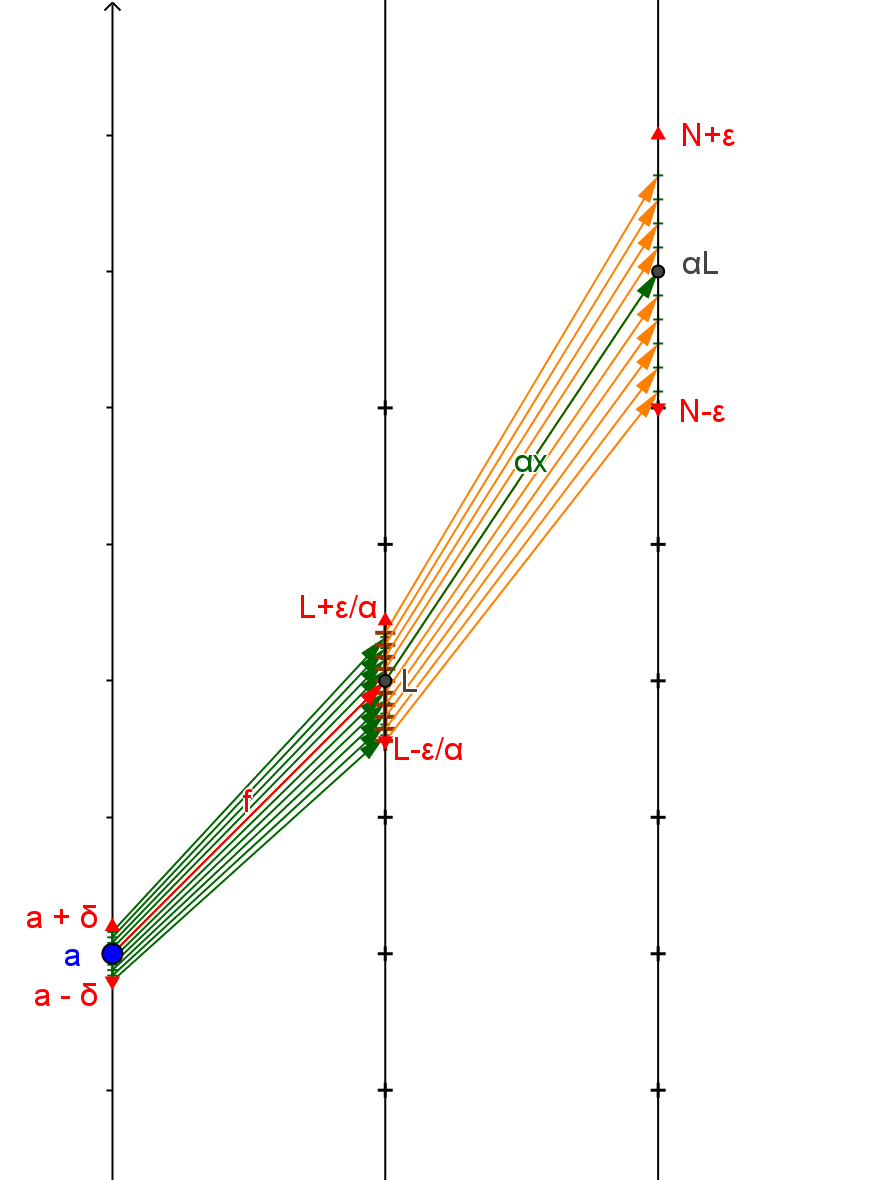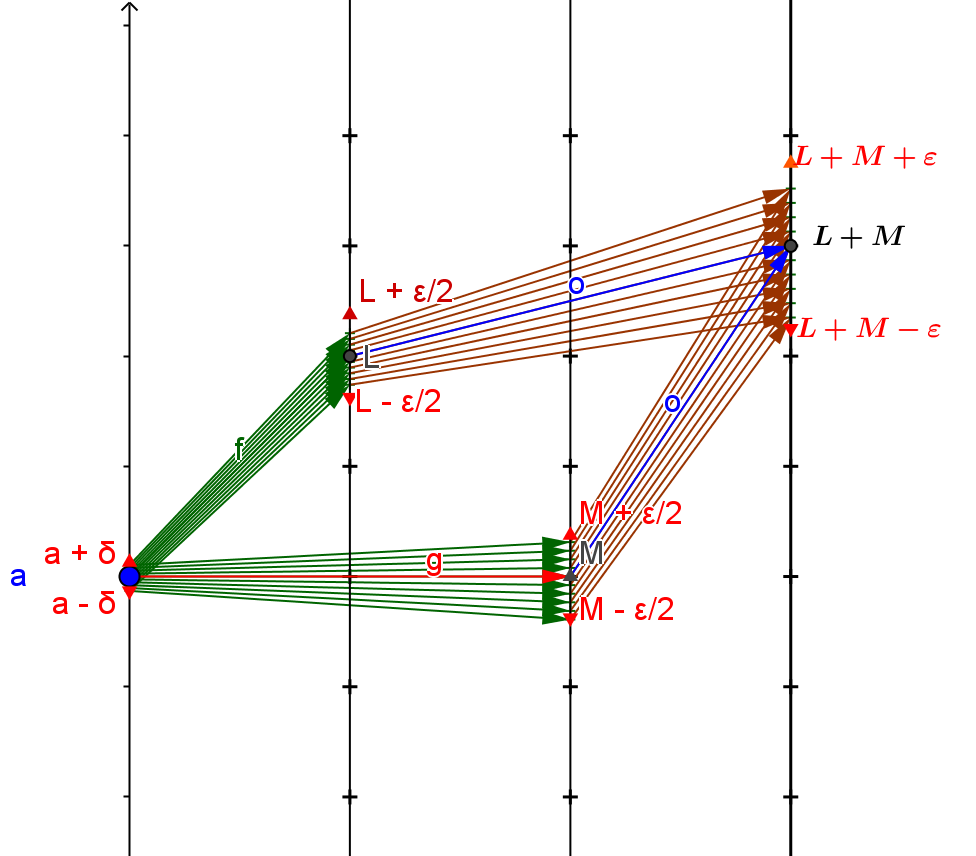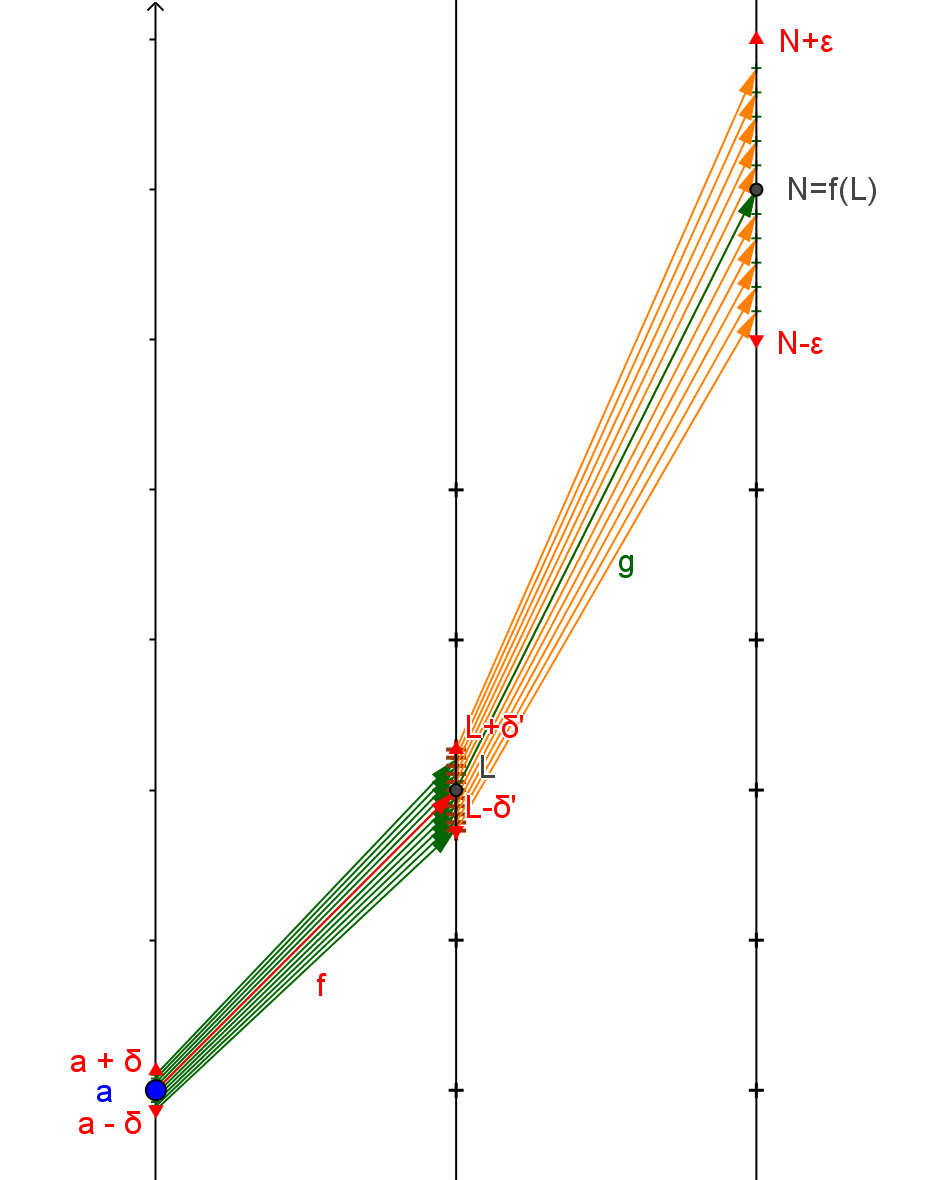Discussion: These results for the calculus of limits seem "obvious"; it makes sense that arithmetic operations should not have a serious effect on approximations.
The proofs of these results are sometimes technically tricky and often not covered in most begining calculus courses- buried in an appendix and left for a course in real analysis .
In this visualization arguments will be given and visualized with mapping diagrams for (i),(ii), and (v).Some Proofs of CCD.CL:
(i)
To show $\lim_{x \to a} \alpha f(x)= \alpha L$ we start with an $\epsilon >0$.
Then consider $\epsilon ' = |\frac{\epsilon}{\alpha}|$.
Since $\lim_{x \to a}f(x)= L$, there is a number $\delta > 0$ where if $a-\delta<x<a+ \delta$ and $x\ne a$ then $L-\epsilon ' < f(x) < L + \epsilon '$.
Now multiplying this inequality by $\alpha$  will either preserve or reverse the inquality but the consequence is that $\alpha L - \epsilon <\alpha f(x) <\alpha L + \epsilon$.

(ii) To show $\lim_{x \to a} f(x) + g(x) = L + M$ we start with an $\epsilon >0$.Then consider $\epsilon ' =\frac{\epsilon}2$.
Since $\lim_{x \to a}f(x)= L$  and $\lim_{x \to a}g(x)= M$ there is a number $\delta > 0$ where if $a-\delta<x<a+ \delta$ and $x\ne a$ then $L-\epsilon ' < f(x) < L + \epsilon '$ and $M-\epsilon ' < g(x) < M + \epsilon '$. Adding these inequalities shows that $L+M- 2\epsilon ' < f(x) +g(x) < L + M + 2 \epsilon '$ or $L+M- \epsilon < f(x) +g(x) < L + M + \epsilon$ . Thus $\lim_{x \to a} f(x) + g(x) = L + M$.
To show $\lim_{x \to a} f(x) - g(x) = L - M$ apply the result just obtained to $\alpha g(x)$ where $\alpha = -1.$

(v) To show $\lim_{x \to a} g(f(x))= N$ we start with an $\epsilon >0$.
Since $\lim_{y \to L} g(y)= N$ and $g(L)=N$, there is a $\delta'>0$ where if  $L-\delta'<y<L+ \delta'$ then $N-\epsilon < g(y) < N + \epsilon$.Then consider $\epsilon ' = \delta '$. Since $\lim_{x \to a}f(x)= L$ there is a $\delta >0$ where if $a-\delta<x<a+ \delta$ and $x\ne a$ then $L-\epsilon ' < f(x) < L + \epsilon '$ or $L-\delta ' < f(x) < L + \delta '$ and so $N-\epsilon < g(f(x)) < N + \epsilon$. Thus $\lim_{x \to a} g(f(x))= N$.

Below is a GeoGebra visualization of this argument using mapping diagrams applied to $f(x)= 2x+1, g(x) = 3x-2, a =1, L=3$, and $M =1$.
To visualize (i) consider the composition of $f(x)$ with $g(x) = \alpha x$, so $g(f(x)) = \alpha f(x)$.

You can change the values of $a, \epsilon$, and $\delta$, as well as the functions $f$ and $g$ to explore the arguments further with other examples
Consider especially the composition with $g(x)=3 x$ for $\alpha = 3$ in  (i).

Martin Flashman, 20 Jan 2018, Created with GeoGebra